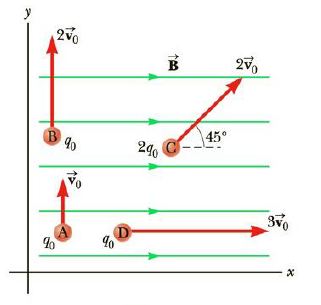Chapter 19, Problem 9CQ

Chapter
Section
Textbook Problem

Figure CQ19.9 shows four positive charges, A, B, C, and D, moving in the xy-plane in the presence of a constant magnetic field. Rank the charges by the magnitude of the magnetic force exerted on them, from largest to smallest. (a) C, D, B, A (b) D, C, B, A (c) A, B, C, D (d) C, B, A, DFigure CQ19.9

To determine

The rank of charges by the magnitude of the magnetic force exerted on them from largest to smallest.

Explanation

The magnetic force on a charged particle is defined as,

F=qvBsinθ

• F is the magnetic force on the charged particle
• q is the charge on the particle
• v is the velocity of the particle
• B is the magnetic field
• θ is the angle between velocity and magnetic field

The magnetic force on charge A is,

FA=q0v0sin90°=q0v0      (1)

The magnetic force on charge B is,

FB=q0(2v0)sin90°=2q0v0      (2)

The magnetic force on charge C

Still sussing out bartleby?

Check out a sample textbook solution.

See a sample solution

The Solution to Your Study Problems

Bartleby provides explanations to thousands of textbook problems written by our experts, many with advanced degrees!

Get Started